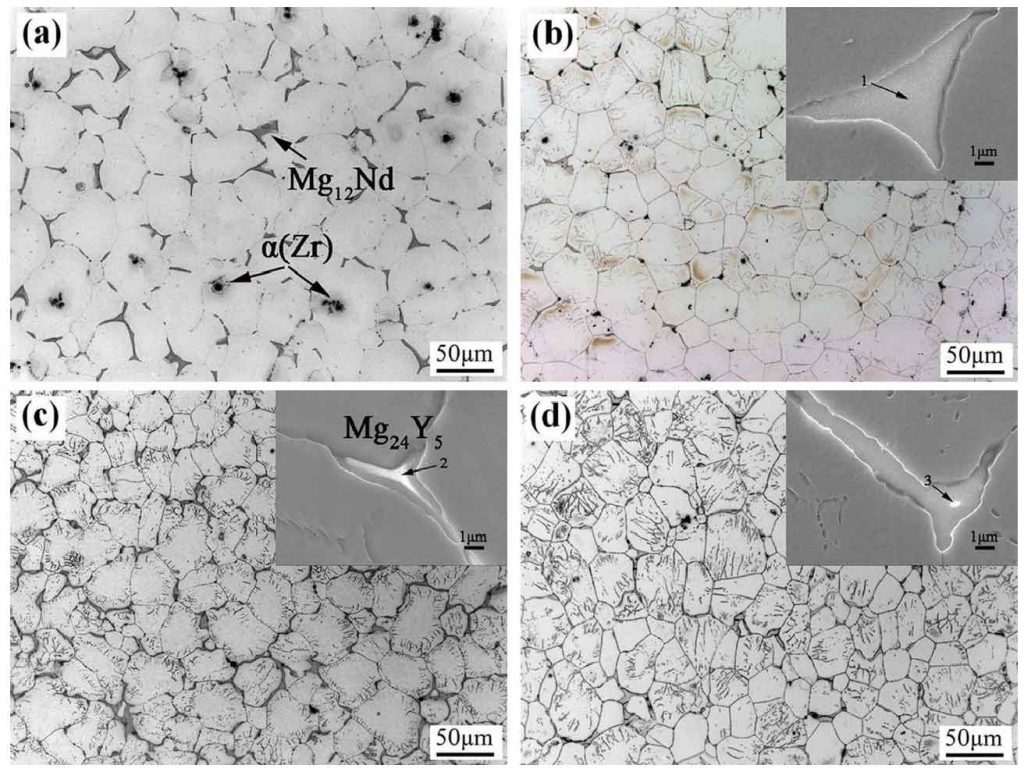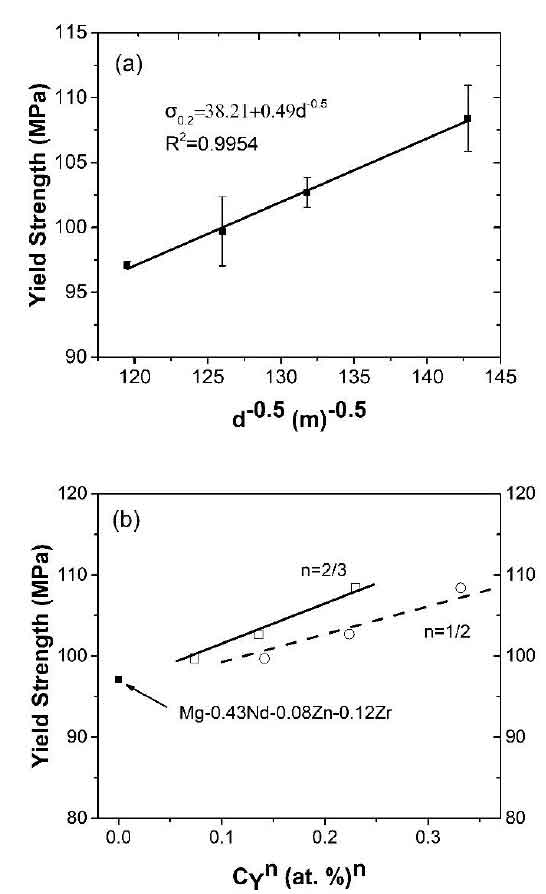# Microstructure and mechanical properties of metal mold casting alloyOptical micrographs of the solution-treated Mg–0.43Nd –xY–0.08Zn–0.11Zr alloys. (a)x=0; (b) x=0.03; (c) x=0.06; (d) x=0.12.

Figure 1 shows the optical structure of Mg – 0.43nd – XY – 0.08zn – 0.11zr alloy after solution treatment. With the increase of Y content from 0 to 0.12%, the grain size of the alloy decreases from 70 μ m to 49 μ M. When y element is not added in the alloy, the eutectic phase of mg12nd almost completely dissolves into α – Mg matrix after solution treatment (Fig. 1a), which can also be seen from the XRD pattern of Fig. 2. In the solid solution alloy containing y, almost all mg24y5 is dissolved in α – Mg matrix, but a small amount of mg12nd phase remains at the intersection of grain boundaries (Fig. 1b, C and D), which can also be obtained from XRD results (Fig. 2). In addition, the gray acicular mg12nd phase (Fig. 3b-d) in the α – Mg grains is almost completely dissolved, and some long rod like phases are found in the alloy.Microstructures of as-cast Mg–0.43Nd –xY–0.08Zn–0.11Zr (at. %) alloys: (a) x=0; (b)x=0.03; (c) x=0.06; (d) x=0.12. The inset in (b), (c) and (d) are the corresponding high-magnification images by SEM.

Figure 4 shows the mechanical properties of the solution mg – 0.43nd – XY – 0.08zn – 0.11zr alloy. Due to grain refinement and solution strengthening of mg24y5 phase, the yield strength and tensile strength of the alloy increase with the increase of Y content. However, due to the existence of brittle mg12nd eutectic phase at the grain boundary, the crack is easy to propagate along the mg12nd eutectic phase and then fracture, resulting in the decrease of elongation from 23.9% to 15.9%. When y content is the highest (0.12 at.), the yield strength of the alloy reaches the maximum value of 108 MPa. In addition, it is found that the yield strength of the alloy increases with y content (at%) (R2 = 0.9949)

The above formula shows that the strengthening rate of Mg – 0.43nd – XY – 0.08zn – 0.11zr alloy is (D σ 02 / DCY) is 101 MPA / at%, which is higher than that of Mg – ynd-0.08zn – 0.12zr alloy (38 MPA / at%) and Mg – 0.52nd – zzn – 0.12zr alloy (50 MPa / at%). The relationship between yield strength and average grain size of mg-nd-zn-y-zr solid solution alloy is shown in Figure 4.

It is well known that the yield strength ys (σ 0.2) and grain size D of the alloy can be described by the following hall Petch relation:

Where k is the strengthening coefficient, σ 0 is the friction stress, σ CRSs is related to the critical shear stress, and σ P is the residual stress. According to Fleischer model and labusch model, n = 1 / 2 or n = 2 / 3. Therefore, the yield strength of solid solution alloy can be expressed as follows:

The data in Figure 5A can be fitted to the following linear relationship (R2 = 0.9954)YS of solution treated Mg–0.43Nd –xY–0.08Zn–0.11Zr alloys as a function of (a) grainsize; (b) CYn.

The above formula shows that σ 0 is about 38.2 MPa and the grain boundary strengthening is 0.49 d-0.5. The strengthening coefficient K is 0.49 MPa M1 / 2, which is larger than that of Mg – ynd zzn – xZr alloy (0.42 MPa M1 / 2). These two values are in agreement with the reported values. Fig. 6-7b shows the relationship between the yield strength value of solid solution mg – 0.43nd – XY – 0.08zn – 0.11zr alloy and CYN based on Akhtar and lightsoonaian models. The fitting result is K y = 34 MPa (at.%) − 1 / 2 or 50 MPa (at.%) − 2 / 3. The existing research results show that for Mg nd Zn Zr alloy, the fitting result of n = 2 / 3 is closer to the experimental result than that of n = 1 / 2. For Mg – 0.43nd – XY – 0.08zn – 0.11zr alloy, when σ CRSs = 5 MPa, σ P = 15MPa, KZN = 40 MPa (at.%) – 2 / 3, knd = 18 MPa (at.%) – 2 / 3.